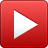# Xavier Jones

## Xavier Jones

•#### Post High School Decisons /* <![CDATA[ */ jQuery(document).ready(function(\$){ \$(function(){ var width = \$(window).innerWidth(); var setwidth = 640; var ratio = 0.75; var height = 480; var link = 'https://www.youtube.com/watch?v=lezdOsP2-qU&width=640&height=480'; if(width < setwidth) { height = Math.floor(width * 0.75); //console.log("device width "+width+", set width "+640+", ratio "+0.75+", new height "+ height); var new_url = wpvl_paramReplace('width', link, width); var new_url = wpvl_paramReplace('height', new_url, height); \$("a#61a4e6a0e2f34").attr('href', new_url); //console.log(new_url); } }); }); /* ]]> */

•#### Post High School Decisions /* <![CDATA[ */ jQuery(document).ready(function(\$){ \$(function(){ var width = \$(window).innerWidth(); var setwidth = 640; var ratio = 0.75; var height = 480; var link = 'https://www.youtube.com/watch?v=KsbZ9SSQf1A&width=640&height=480'; if(width < setwidth) { height = Math.floor(width * 0.75); //console.log("device width "+width+", set width "+640+", ratio "+0.75+", new height "+ height); var new_url = wpvl_paramReplace('width', link, width); var new_url = wpvl_paramReplace('height', new_url, height); \$("a#61a4e6a0e3056").attr('href', new_url); //console.log(new_url); } }); }); /* ]]> */

•#### Working and Football Disappointment /* <![CDATA[ */ jQuery(document).ready(function(\$){ \$(function(){ var width = \$(window).innerWidth(); var setwidth = 640; var ratio = 0.75; var height = 480; var link = 'https://www.youtube.com/watch?v=BSOTRlUmZlA&width=640&height=480'; if(width < setwidth) { height = Math.floor(width * 0.75); //console.log("device width "+width+", set width "+640+", ratio "+0.75+", new height "+ height); var new_url = wpvl_paramReplace('width', link, width); var new_url = wpvl_paramReplace('height', new_url, height); \$("a#61a4e6a0e3175").attr('href', new_url); //console.log(new_url); } }); }); /* ]]> */

•#### Sisters Raised Me /* <![CDATA[ */ jQuery(document).ready(function(\$){ \$(function(){ var width = \$(window).innerWidth(); var setwidth = 640; var ratio = 0.75; var height = 480; var link = 'https://www.youtube.com/watch?v=3uZs9fPvvdE&width=640&height=480'; if(width < setwidth) { height = Math.floor(width * 0.75); //console.log("device width "+width+", set width "+640+", ratio "+0.75+", new height "+ height); var new_url = wpvl_paramReplace('width', link, width); var new_url = wpvl_paramReplace('height', new_url, height); \$("a#61a4e6a0e3273").attr('href', new_url); //console.log(new_url); } }); }); /* ]]> */

•#### Taking Care of Mom /* <![CDATA[ */ jQuery(document).ready(function(\$){ \$(function(){ var width = \$(window).innerWidth(); var setwidth = 640; var ratio = 0.75; var height = 480; var link = 'https://www.youtube.com/watch?v=kKM1DfKPIg0&width=640&height=480'; if(width < setwidth) { height = Math.floor(width * 0.75); //console.log("device width "+width+", set width "+640+", ratio "+0.75+", new height "+ height); var new_url = wpvl_paramReplace('width', link, width); var new_url = wpvl_paramReplace('height', new_url, height); \$("a#61a4e6a0e3366").attr('href', new_url); //console.log(new_url); } }); }); /* ]]> */

•#### Growing Up in Kent County /* <![CDATA[ */ jQuery(document).ready(function(\$){ \$(function(){ var width = \$(window).innerWidth(); var setwidth = 640; var ratio = 0.75; var height = 480; var link = 'https://www.youtube.com/watch?v=uNge9fDChhQ&width=640&height=480'; if(width < setwidth) { height = Math.floor(width * 0.75); //console.log("device width "+width+", set width "+640+", ratio "+0.75+", new height "+ height); var new_url = wpvl_paramReplace('width', link, width); var new_url = wpvl_paramReplace('height', new_url, height); \$("a#61a4e6a0e34ac").attr('href', new_url); //console.log(new_url); } }); }); /* ]]> */

•#### Galena Funeral Home /* <![CDATA[ */ jQuery(document).ready(function(\$){ \$(function(){ var width = \$(window).innerWidth(); var setwidth = 640; var ratio = 0.75; var height = 480; var link = 'https://www.youtube.com/watch?v=oI6jU-6faRc&width=640&height=480'; if(width < setwidth) { height = Math.floor(width * 0.75); //console.log("device width "+width+", set width "+640+", ratio "+0.75+", new height "+ height); var new_url = wpvl_paramReplace('width', link, width); var new_url = wpvl_paramReplace('height', new_url, height); \$("a#61a4e6a0e35a9").attr('href', new_url); //console.log(new_url); } }); }); /* ]]> */

•#### First Heartbreak /* <![CDATA[ */ jQuery(document).ready(function(\$){ \$(function(){ var width = \$(window).innerWidth(); var setwidth = 640; var ratio = 0.75; var height = 480; var link = 'https://www.youtube.com/watch?v=-vTFy7Weqkc&width=640&height=480'; if(width < setwidth) { height = Math.floor(width * 0.75); //console.log("device width "+width+", set width "+640+", ratio "+0.75+", new height "+ height); var new_url = wpvl_paramReplace('width', link, width); var new_url = wpvl_paramReplace('height', new_url, height); \$("a#61a4e6a0e36b1").attr('href', new_url); //console.log(new_url); } }); }); /* ]]> */

Interview and video production by Gordon Wallace